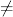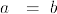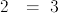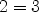login  home  contents  what's new  discussion  bug reports help  links  subscribe  changes  refresh  edit

# Edit detail for SandBoxInequation revision 1 of 11

 1 2 3 4 5 6 7 8 9 10 11 Editor: Bill Page Time: 2008/06/09 19:55:32 GMT-7 Note: Inequations as mathematical objects

changed:
-
)abbrev domain NE Inequation
--FOR THE BENEFIT  OF LIBAX0 GENERATION
++ Author: Bill Page
++ Based on: Equation by Stephen M. Watt, enhancements by Johannes Grabmeier
++ Date Created: June 2008
++ Basic Operations: ~=
++ Related Domains: Equation
++ Also See:
++ AMS Classifications:
++ Keywords: inequation
++ Examples:
++ References:
++ Description:
++   Inequations as mathematical objects.  All properties of the basis domain,
++   e.g. being an abelian group are carried over the equation domain, by
++   performing the structural operations on the left and on the
++   right hand side.
--   The interpreter translates "~=" to "inequation".  Otherwise, it will
--   find a modemap for "~=" in the domain of the arguments.

Inequation(S: Type): public == private where
public ==> Type with
"~=": (S, S) -> $++ a~=b creates an inequation. inequation: (S, S) ->$
++ inequation(a,b) creates an inequation.
swap: $->$
++ swap(eq) interchanges left and right hand side of inequation eq.
lhs: $-> S ++ lhs(eqn) returns the left hand side of inequation eqn. rhs:$ -> S
++ rhs(eqn) returns the right hand side of inequation eqn.
map: (S -> S, $) ->$
++ map(f,eqn) constructs a new inequation by applying f to both
++ sides of eqn. (f must be an injection)
if S has InnerEvalable(Symbol,S) then
InnerEvalable(Symbol,S)
if S has SetCategory then
SetCategory
CoercibleTo Boolean
if S has Evalable(S) then
eval: ($, Equation S) ->$
++ eval(eqn, x=f) replaces x by f in inequation eqn.
eval: ($, List Equation S) ->$
++ eval(eqn, [x1=v1, ... xn=vn]) replaces xi by vi in inequation eqn.
if S has AbelianSemiGroup then
AbelianSemiGroup
"+": (S, $) ->$
++ x+eqn produces a new inequation by adding x to both sides of
++ inequation eqn.
"+": ($, S) ->$
++ eqn+x produces a new inequation by adding x to both sides of
++ inequation eqn.
if S has AbelianGroup then
AbelianGroup
leftZero : $->$
++ leftZero(eq) subtracts the left hand side.
rightZero : $->$
++ rightZero(eq) subtracts the right hand side.
"-": (S, $) ->$
++ x-eqn produces a new equation by subtracting both sides of
++ equation eqn from x.
"-": ($, S) ->$
++ eqn-x produces a new equation by subtracting x from  both sides of
++ equation eqn.
if S has SemiGroup then
SemiGroup
"*": (S, $) ->$
++ x*eqn produces a new equation by multiplying both sides of
++ equation eqn by x.
"*": ($, S) ->$
++ eqn*x produces a new equation by multiplying both sides of
++ equation eqn by x.
if S has Monoid then
Monoid
leftOne : $-> Union($,"failed")
++ leftOne(eq) divides by the left hand side, if possible.
rightOne : $-> Union($,"failed")
++ rightOne(eq) divides by the right hand side, if possible.
if S has Group then
Group
leftOne : $-> Union($,"failed")
++ leftOne(eq) divides by the left hand side.
rightOne : $-> Union($,"failed")
++ rightOne(eq) divides by the right hand side.
if S has Ring then
Ring
BiModule(S,S)
if S has CommutativeRing then
Module(S)
--Algebra(S)
if S has IntegralDomain then
factorAndSplit : $-> List$
++ factorAndSplit(eq) make the right hand side 0 and
++ factors the new left hand side. Each factor is equated
++ to 0 and put into the resulting list without repetitions.
if S has PartialDifferentialRing(Symbol) then
PartialDifferentialRing(Symbol)
if S has Field then
VectorSpace(S)
"/": ($,$) -> $++ e1/e2 produces a new equation by dividing the left and right ++ hand sides of equations e1 and e2. inv:$ -> $++ inv(x) returns the multiplicative inverse of x. if S has ExpressionSpace then subst: ($, $) ->$
++ subst(eq1,eq2) substitutes eq2 into both sides of eq1
++ the lhs of eq2 should be a kernel

Rep := Record(lhs: S, rhs: S)
eq1,eq2: $s : S if S has IntegralDomain then factorAndSplit eq == (S has factor : S -> Factored S) => eq0 := rightZero eq [inequation(rcf.factor,0) for rcf in factors factor lhs eq0] [eq] l:S ~= r:S == [l, r] inequation(l, r) == [l, r] -- hack! See comment above. lhs eqn == eqn.lhs rhs eqn == eqn.rhs swap eqn == [rhs eqn, lhs eqn] map(fn, eqn) == inequation(fn(eqn.lhs), fn(eqn.rhs)) if S has InnerEvalable(Symbol,S) then s:Symbol ls:List Symbol x:S lx:List S eval(eqn,s,x) == eval(eqn.lhs,s,x) ~= eval(eqn.rhs,s,x) eval(eqn,ls,lx) == eval(eqn.lhs,ls,lx) ~= eval(eqn.rhs,ls,lx) if S has Evalable(S) then eval(eqn1:$, eqn2:Equation S):$== eval(eqn1.lhs, eqn2) ~= eval(eqn1.rhs, eqn2) eval(eqn1:$, leqn2:List Equation S):$== eval(eqn1.lhs, leqn2) ~= eval(eqn1.rhs, leqn2) if S has SetCategory then eq1 = eq2 == (eq1.lhs = eq2.lhs)@Boolean and (eq1.rhs = eq2.rhs)@Boolean coerce(eqn:$):OutputForm == blankSeparate([eqn.lhs::OutputForm, "~=", eqn.rhs::OutputForm])$OutputForm coerce(eqn:$):Boolean == eqn.lhs ~= eqn.rhs
if S has AbelianSemiGroup then
eq1 + eq2 == eq1.lhs + eq2.lhs ~= eq1.rhs + eq2.rhs
s + eq2 == [s,s] + eq2
eq1 + s == eq1 + [s,s]
if S has AbelianGroup then
- eq == (- lhs eq) ~= (-rhs eq)
s - eq2 == [s,s] - eq2
eq1 - s == eq1 - [s,s]
leftZero eq == 0 ~= rhs eq - lhs eq
rightZero eq == lhs eq - rhs eq ~= 0
0 == inequation(0$S,0$S)
eq1 - eq2 == eq1.lhs - eq2.lhs ~= eq1.rhs - eq2.rhs
if S has SemiGroup then
eq1:$* eq2:$ == eq1.lhs * eq2.lhs ~= eq1.rhs * eq2.rhs
l:S   * eqn:$== l * eqn.lhs ~= l * eqn.rhs l:S * eqn:$  ==  l * eqn.lhs    ~=    l * eqn.rhs
eqn:$* l:S == eqn.lhs * l ~= eqn.rhs * l -- We have to be a bit careful here: raising to a +ve integer is OK -- (since it's the equivalent of repeated multiplication) -- but other powers may cause contradictions -- Watch what else you add here! JHD 2/Aug 1990 if S has Monoid then 1 == inequation(1$S,1$S) recip eq == (lh := recip lhs eq) case "failed" => "failed" (rh := recip rhs eq) case "failed" => "failed" [lh :: S, rh :: S] leftOne eq == (re := recip lhs eq) case "failed" => "failed" 1 ~= rhs eq * re rightOne eq == (re := recip rhs eq) case "failed" => "failed" lhs eq * re ~= 1 if S has Group then inv eq == [inv lhs eq, inv rhs eq] leftOne eq == 1 ~= rhs eq * inv rhs eq rightOne eq == lhs eq * inv rhs eq ~= 1 if S has Ring then characteristic() == characteristic()$S
i:Integer * eq:$== (i::S) * eq if S has IntegralDomain then factorAndSplit eq == (S has factor : S -> Factored S) => eq0 := rightZero eq [inequation(rcf.factor,0) for rcf in factors factor lhs eq0] (S has Polynomial Integer) => eq0 := rightZero eq MF ==> MultivariateFactorize(Symbol, IndexedExponents Symbol, _ Integer, Polynomial Integer) p : Polynomial Integer := (lhs eq0) pretend Polynomial Integer [inequation((rcf.factor) pretend S,0) for rcf in factors factor(p)$MF]
[eq]
if S has PartialDifferentialRing(Symbol) then
differentiate(eq:$, sym:Symbol):$ ==
[differentiate(lhs eq, sym), differentiate(rhs eq, sym)]
if S has Field then
dimension() == 2 :: CardinalNumber
eq1:$/ eq2:$ == eq1.lhs / eq2.lhs ~= eq1.rhs / eq2.rhs
inv eq == [inv lhs eq, inv rhs eq]
if S has ExpressionSpace then
subst(eq1,eq2) ==
eq3 := eq2 pretend Equation S
[subst(lhs eq1,eq3),subst(rhs eq1,eq3)]

It works but the LaTeX output does not display $\ne$
\begin{axiom}
)set output tex on
)set output algebra on
inequation(a,b)
inequation(2,3)
equation(2,3)
\end{axiom}



spad)abbrev domain NE Inequation
--FOR THE BENEFIT  OF LIBAX0 GENERATION
++ Author: Bill Page
++ Based on: Equation by Stephen M. Watt, enhancements by Johannes Grabmeier
++ Date Created: June 2008
++ Basic Operations: ~=
++ Related Domains: Equation
++ Also See:
++ AMS Classifications:
++ Keywords: inequation
++ Examples:
++ References:
++ Description:
++   Inequations as mathematical objects.  All properties of the basis domain,
++   e.g. being an abelian group are carried over the equation domain, by
++   performing the structural operations on the left and on the
++   right hand side.
--   The interpreter translates "~=" to "inequation".  Otherwise, it will
--   find a modemap for "~=" in the domain of the arguments.
Inequation(S: Type): public == private where
public ==> Type with
"~=": (S, S) -> $++ a~=b creates an inequation. inequation: (S, S) ->$
++ inequation(a,b) creates an inequation.
swap: $->$
++ swap(eq) interchanges left and right hand side of inequation eq.
lhs: $-> S ++ lhs(eqn) returns the left hand side of inequation eqn. rhs:$ -> S
++ rhs(eqn) returns the right hand side of inequation eqn.
map: (S -> S, $) ->$
++ map(f,eqn) constructs a new inequation by applying f to both
++ sides of eqn. (f must be an injection)
if S has InnerEvalable(Symbol,S) then
InnerEvalable(Symbol,S)
if S has SetCategory then
SetCategory
CoercibleTo Boolean
if S has Evalable(S) then
eval: ($, Equation S) ->$
++ eval(eqn, x=f) replaces x by f in inequation eqn.
eval: ($, List Equation S) ->$
++ eval(eqn, [x1=v1, ... xn=vn]) replaces xi by vi in inequation eqn.
if S has AbelianSemiGroup then
AbelianSemiGroup
"+": (S, $) ->$
++ x+eqn produces a new inequation by adding x to both sides of
++ inequation eqn.
"+": ($, S) ->$
++ eqn+x produces a new inequation by adding x to both sides of
++ inequation eqn.
if S has AbelianGroup then
AbelianGroup
leftZero : $->$
++ leftZero(eq) subtracts the left hand side.
rightZero : $->$
++ rightZero(eq) subtracts the right hand side.
"-": (S, $) ->$
++ x-eqn produces a new equation by subtracting both sides of
++ equation eqn from x.
"-": ($, S) ->$
++ eqn-x produces a new equation by subtracting x from  both sides of
++ equation eqn.
if S has SemiGroup then
SemiGroup
"*": (S, $) ->$
++ x*eqn produces a new equation by multiplying both sides of
++ equation eqn by x.
"*": ($, S) ->$
++ eqn*x produces a new equation by multiplying both sides of
++ equation eqn by x.
if S has Monoid then
Monoid
leftOne : $-> Union($,"failed")
++ leftOne(eq) divides by the left hand side, if possible.
rightOne : $-> Union($,"failed")
++ rightOne(eq) divides by the right hand side, if possible.
if S has Group then
Group
leftOne : $-> Union($,"failed")
++ leftOne(eq) divides by the left hand side.
rightOne : $-> Union($,"failed")
++ rightOne(eq) divides by the right hand side.
if S has Ring then
Ring
BiModule(S,S)
if S has CommutativeRing then
Module(S)
--Algebra(S)
if S has IntegralDomain then
factorAndSplit : $-> List$
++ factorAndSplit(eq) make the right hand side 0 and
++ factors the new left hand side. Each factor is equated
++ to 0 and put into the resulting list without repetitions.
if S has PartialDifferentialRing(Symbol) then
PartialDifferentialRing(Symbol)
if S has Field then
VectorSpace(S)
"/": ($,$) -> $++ e1/e2 produces a new equation by dividing the left and right ++ hand sides of equations e1 and e2. inv:$ -> $++ inv(x) returns the multiplicative inverse of x. if S has ExpressionSpace then subst: ($, $) ->$
++ subst(eq1,eq2) substitutes eq2 into both sides of eq1
++ the lhs of eq2 should be a kernel
Rep := Record(lhs: S, rhs: S)
eq1,eq2: $s : S if S has IntegralDomain then factorAndSplit eq == (S has factor : S -> Factored S) => eq0 := rightZero eq [inequation(rcf.factor,0) for rcf in factors factor lhs eq0] [eq] l:S ~= r:S == [l, r] inequation(l, r) == [l, r] -- hack! See comment above. lhs eqn == eqn.lhs rhs eqn == eqn.rhs swap eqn == [rhs eqn, lhs eqn] map(fn, eqn) == inequation(fn(eqn.lhs), fn(eqn.rhs)) if S has InnerEvalable(Symbol,S) then s:Symbol ls:List Symbol x:S lx:List S eval(eqn,s,x) == eval(eqn.lhs,s,x) ~= eval(eqn.rhs,s,x) eval(eqn,ls,lx) == eval(eqn.lhs,ls,lx) ~= eval(eqn.rhs,ls,lx) if S has Evalable(S) then eval(eqn1:$, eqn2:Equation S):$== eval(eqn1.lhs, eqn2) ~= eval(eqn1.rhs, eqn2) eval(eqn1:$, leqn2:List Equation S):$== eval(eqn1.lhs, leqn2) ~= eval(eqn1.rhs, leqn2) if S has SetCategory then eq1 = eq2 == (eq1.lhs = eq2.lhs)@Boolean and (eq1.rhs = eq2.rhs)@Boolean coerce(eqn:$):OutputForm == blankSeparate([eqn.lhs::OutputForm, "~=", eqn.rhs::OutputForm])$OutputForm coerce(eqn:$):Boolean == eqn.lhs ~= eqn.rhs
if S has AbelianSemiGroup then
eq1 + eq2 == eq1.lhs + eq2.lhs ~= eq1.rhs + eq2.rhs
s + eq2 == [s,s] + eq2
eq1 + s == eq1 + [s,s]
if S has AbelianGroup then
- eq == (- lhs eq) ~= (-rhs eq)
s - eq2 == [s,s] - eq2
eq1 - s == eq1 - [s,s]
leftZero eq == 0 ~= rhs eq - lhs eq
rightZero eq == lhs eq - rhs eq ~= 0
0 == inequation(0$S,0$S)
eq1 - eq2 == eq1.lhs - eq2.lhs ~= eq1.rhs - eq2.rhs
if S has SemiGroup then
eq1:$* eq2:$ == eq1.lhs * eq2.lhs ~= eq1.rhs * eq2.rhs
l:S   * eqn:$== l * eqn.lhs ~= l * eqn.rhs l:S * eqn:$  ==  l * eqn.lhs    ~=    l * eqn.rhs
eqn:$* l:S == eqn.lhs * l ~= eqn.rhs * l -- We have to be a bit careful here: raising to a +ve integer is OK -- (since it's the equivalent of repeated multiplication) -- but other powers may cause contradictions -- Watch what else you add here! JHD 2/Aug 1990 if S has Monoid then 1 == inequation(1$S,1$S) recip eq == (lh := recip lhs eq) case "failed" => "failed" (rh := recip rhs eq) case "failed" => "failed" [lh :: S, rh :: S] leftOne eq == (re := recip lhs eq) case "failed" => "failed" 1 ~= rhs eq * re rightOne eq == (re := recip rhs eq) case "failed" => "failed" lhs eq * re ~= 1 if S has Group then inv eq == [inv lhs eq, inv rhs eq] leftOne eq == 1 ~= rhs eq * inv rhs eq rightOne eq == lhs eq * inv rhs eq ~= 1 if S has Ring then characteristic() == characteristic()$S
i:Integer * eq:$== (i::S) * eq if S has IntegralDomain then factorAndSplit eq == (S has factor : S -> Factored S) => eq0 := rightZero eq [inequation(rcf.factor,0) for rcf in factors factor lhs eq0] (S has Polynomial Integer) => eq0 := rightZero eq MF ==> MultivariateFactorize(Symbol, IndexedExponents Symbol, _ Integer, Polynomial Integer) p : Polynomial Integer := (lhs eq0) pretend Polynomial Integer [inequation((rcf.factor) pretend S,0) for rcf in factors factor(p)$MF]
[eq]
if S has PartialDifferentialRing(Symbol) then
differentiate(eq:$, sym:Symbol):$ ==
[differentiate(lhs eq, sym), differentiate(rhs eq, sym)]
if S has Field then
dimension() == 2 :: CardinalNumber
eq1:$/ eq2:$ == eq1.lhs / eq2.lhs ~= eq1.rhs / eq2.rhs
inv eq == [inv lhs eq, inv rhs eq]
if S has ExpressionSpace then
subst(eq1,eq2) ==
eq3 := eq2 pretend Equation S
[subst(lhs eq1,eq3),subst(rhs eq1,eq3)]
   Compiling FriCAS source code from file
old system compiler.
NE abbreviates domain Inequation
processing macro definition public ==> -- the constructor category
processing macro definition private ==> -- the constructor capsule
------------------------------------------------------------------------
initializing NRLIB NE for Inequation
compiling into NRLIB NE
****** Domain: S already in scope
augmenting S: (IntegralDomain)
augmenting $: (SIGNATURE$ factorAndSplit ((List $)$))
compiling exported factorAndSplit : $-> List$
augmenting S: (SIGNATURE S factor ((Factored S) S))
Time: 0.10 SEC.
compiling exported ~= : (S,S) -> $NE;~=;2S$;2 is replaced by CONS
Time: 0 SEC.
compiling exported inequation : (S,S) -> $NE;inequation;2S$;3 is replaced by CONS
Time: 0 SEC.
compiling exported lhs : $-> S NE;lhs;$S;4 is replaced by QCAR
Time: 0 SEC.
compiling exported rhs : $-> S NE;rhs;$S;5 is replaced by QCDR
Time: 0 SEC.
compiling exported swap : $->$
Time: 0 SEC.
compiling exported map : (S -> S,$) ->$
Time: 0 SEC.
****** Domain: S already in scope
augmenting S: (InnerEvalable (Symbol) S)
compiling exported eval : ($,Symbol,S) ->$
Time: 0.01 SEC.
compiling exported eval : ($,List Symbol,List S) ->$
Time: 0.01 SEC.
****** Domain: S already in scope
augmenting S: (Evalable S)
compiling exported eval : ($,Equation S) ->$
Time: 0 SEC.
compiling exported eval : ($,List Equation S) ->$
Time: 0 SEC.
****** Domain: S already in scope
augmenting S: (SetCategory)
compiling exported = : ($,$) -> Boolean
Time: 0.07 SEC.
compiling exported coerce : $-> OutputForm Time: 0 SEC. compiling exported coerce :$ -> Boolean
Time: 0.01 SEC.
****** Domain: S already in scope
augmenting S: (AbelianSemiGroup)
augmenting $: (SIGNATURE$ + ($S$))
augmenting $: (SIGNATURE$ + ( S))
compiling exported + : ($,$) -> $Time: 0 SEC. compiling exported + : (S,$) -> $Time: 0 SEC. compiling exported + : ($,S) -> $Time: 0 SEC. ****** Domain: S already in scope augmenting S: (AbelianGroup) augmenting$: (SIGNATURE $leftZero ($ $)) augmenting$: (SIGNATURE $rightZero ($ $)) augmenting$: (SIGNATURE $- ($ S $)) augmenting$: (SIGNATURE $- ($ $S)) compiling exported - :$ -> $Time: 0.01 SEC. compiling exported - : (S,$) -> $Time: 0 SEC. compiling exported - : ($,S) -> $Time: 0 SEC. compiling exported leftZero :$ -> $Time: 0 SEC. compiling exported rightZero :$ -> $Time: 0 SEC. compiling exported Zero : () ->$
Time: 0 SEC.
compiling exported - : ($,$) -> $Time: 0 SEC. ****** Domain: S already in scope augmenting S: (SemiGroup) augmenting$: (SIGNATURE $* ($ S $)) augmenting$: (SIGNATURE $* ($ $S)) compiling exported * : ($,$) ->$
Time: 0.01 SEC.
compiling exported * : (S,$) ->$
Time: 0 SEC.
compiling exported * : (S,$) ->$
Time: 0 SEC.
compiling exported * : ($,S) ->$
Time: 0 SEC.
****** Domain: S already in scope
augmenting S: (Monoid)
augmenting $: (SIGNATURE$ leftOne ((Union $failed)$))
augmenting $: (SIGNATURE$ rightOne ((Union $failed)$))
compiling exported One : () -> $Time: 0 SEC. compiling exported recip :$ -> Union($,failed) Time: 0 SEC. compiling exported leftOne :$ -> Union($,failed) Time: 0.01 SEC. compiling exported rightOne :$ -> Union($,failed) Time: 0 SEC. ****** Domain: S already in scope augmenting S: (Group) augmenting$: (SIGNATURE $leftOne ((Union$ failed) $)) augmenting$: (SIGNATURE $rightOne ((Union$ failed) $)) compiling exported inv :$ -> $Time: 0.01 SEC. compiling exported leftOne :$ -> Union($,failed) Time: 0 SEC. compiling exported rightOne :$ -> Union($,failed) Time: 0 SEC. ****** Domain: S already in scope augmenting S: (Ring) compiling exported characteristic : () -> NonNegativeInteger Time: 0.06 SEC. compiling exported * : (Integer,$) -> $Time: 0 SEC. ****** Domain: S already in scope augmenting S: (IntegralDomain) augmenting$: (SIGNATURE $factorAndSplit ((List$) $)) compiling exported factorAndSplit :$ -> List $augmenting S: (SIGNATURE S factor ((Factored S) S)) extension of ##1 to (Polynomial (Integer)) ignored processing macro definition MF ==> MultivariateFactorize(Symbol,IndexedExponents Symbol,Integer,Polynomial Integer) Time: 0.18 SEC. ****** Domain: S already in scope augmenting S: (PartialDifferentialRing (Symbol)) compiling exported differentiate : ($,Symbol) -> $Time: 0 SEC. ****** Domain: S already in scope augmenting S: (Field) augmenting$: (SIGNATURE $/ ($ ))
augmenting $: (SIGNATURE$ inv ())
compiling exported dimension : () -> CardinalNumber
Time: 0.02 SEC.
compiling exported / : ($,$) -> $Time: 0 SEC. compiling exported inv :$ -> $Time: 0 SEC. ****** Domain: S already in scope augmenting S: (ExpressionSpace) augmenting$: (SIGNATURE $subst ($ ))
compiling exported subst : ($,$) -> $Time: 0 SEC. ****** Domain: S already in scope augmenting S: (Evalable S) ****** Domain: S already in scope augmenting S: (SetCategory) augmenting$: (SIGNATURE $eval ($ $(Equation S))) augmenting$: (SIGNATURE $eval ($ $(List (Equation S)))) ****** Domain: S already in scope augmenting S: (AbelianGroup) augmenting$: (SIGNATURE $leftZero ($ $)) augmenting$: (SIGNATURE $rightZero ($ $)) augmenting$: (SIGNATURE $- ($ S $)) augmenting$: (SIGNATURE $- ($ $S)) ****** Domain: S already in scope augmenting S: (Field) augmenting$: (SIGNATURE $/ ($ ))
augmenting $: (SIGNATURE$ inv ())
****** Domain: S already in scope
augmenting S: (AbelianGroup)
augmenting $: (SIGNATURE$ leftZero ())
augmenting $: (SIGNATURE$ rightZero ())
augmenting $: (SIGNATURE$ - ($S$))
augmenting $: (SIGNATURE$ - ( S))
****** Domain: S already in scope
augmenting S: (AbelianSemiGroup)
augmenting $: (SIGNATURE$ + ($S$))
augmenting $: (SIGNATURE$ + ( S))
****** Domain: S already in scope
augmenting S: (ExpressionSpace)
augmenting $: (SIGNATURE$ subst ( $)) ****** Domain: S already in scope augmenting S: (Field) augmenting$: (SIGNATURE $/ ($ ))
augmenting $: (SIGNATURE$ inv ())
****** Domain: S already in scope
augmenting S: (Group)
augmenting $: (SIGNATURE$ leftOne ((Union $failed)$))
augmenting $: (SIGNATURE$ rightOne ((Union $failed)$))
****** Domain: S already in scope
augmenting S: (InnerEvalable (Symbol) S)
****** Domain: S already in scope
augmenting S: (IntegralDomain)
augmenting $: (SIGNATURE$ factorAndSplit ((List $)$))
****** Domain: S already in scope
augmenting S: (Monoid)
augmenting $: (SIGNATURE$ leftOne ((Union $failed)$))
augmenting $: (SIGNATURE$ rightOne ((Union $failed)$))
****** Domain: S already in scope
augmenting S: (PartialDifferentialRing (Symbol))
****** Domain: S already in scope
augmenting S: (Ring)
****** Domain: S already in scope
augmenting S: (SemiGroup)
augmenting $: (SIGNATURE$ * ($S$))
augmenting $: (SIGNATURE$ * ( S))
****** Domain: S already in scope
augmenting S: (SetCategory)
(time taken in buildFunctor:  3)
;;;     ***       |Inequation| REDEFINED
;;;     ***       |Inequation| REDEFINED
Time: 0.14 SEC.
Semantic Errors:
 factorAndSplit:  rcf has two modes:
Warnings:
 factorAndSplit: not known that (IntegralDomain) is of mode (CATEGORY domain (SIGNATURE factorAndSplit ((List $)$)))
 factorAndSplit: not known that (IntegralDomain) is of mode (CATEGORY S (SIGNATURE factor ((Factored S) S)))
Cumulative Statistics for Constructor Inequation
Time: 0.64 seconds
finalizing NRLIB NE
Processing Inequation for Browser database:
--------(~= ($S S))--------- --------(inequation ($ S S))---------
--------(swap ())---------
--------(lhs (S $))--------- --------(rhs (S$))---------
--------(map ($(Mapping S S)$))---------
--------(eval ( (Equation S)))---------
--------(eval ( (List (Equation S))))---------
--------(+ ($S$))---------
--------(+ ( S))---------
--------(leftZero ())---------
--------(rightZero ())---------
--------(- ($S$))---------
--------(- ( S))---------
--------(* ($S$))---------
--------(* ( S))---------
--------(leftOne ((Union $failed)$))---------
--------(rightOne ((Union $failed)$))---------
--------(leftOne ((Union $failed)$))---------
--------(rightOne ((Union $failed)$))---------
--------(factorAndSplit ((List $)$))---------
--------(/ ( $))--------- --------(inv ($ $))--------- --------(subst ($ ))---------
--------constructor---------
; (DEFUN |Inequation;| ...) is being compiled.
;; The variable IDENTITY is undefined.
;; The compiler will assume this variable is a global.
------------------------------------------------------------------------
Inequation is now explicitly exposed in frame initial
Inequation will be automatically loaded when needed from
/var/zope2/var/LatexWiki/NE.NRLIB/code

It works but the LaTeX? output does not displayaxiom)set output tex on
)set output algebra on
inequation(a,b)
(1)  a ~= b(1)
Type: Inequation Symbol
axiominequation(2,3)
(2)  2 ~= 3(2)
Type: Inequation PositiveInteger?
axiomequation(2,3)
(3)  2= 3(3)
Type: Equation PositiveInteger?